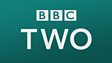This clip shows how to easily subtract tens and near tens. Forty four apples are picked and loaded into a pram so that they can be sold. Ten are removed because they are bruised, and a further 11 are removed because they are maggoty. The main character subtracts 10 from 44. He then takes us through the strategy for subtracting 11 by taking away 10 and then 1. After this, 9 more apples fall out of the pram. The character works out how many he has left by subtracting 10 and adding 1 back. A secondary character eats the apples, so the main character also needs to subtract 1 from various numbers.This clip is from:
Numbertime, Addition and Subtraction: Two step subtraction
First broadcast:
26 November 2001

Recap on the clip, pointing out to the pupils that 11 or 9 were subtracted easily by the character because he could subtract 10. Give the children some quick fire subtraction questions to practise. Move on to subtract 11 by showing the children on the board that it is very similar to subtract 10 but one more is also subtracted. Give the pupils some practice at this and ensure they are secure in this before moving on to subtracting 9 from various two-digit numbers. A hundred number square will provide a visual aid to this.